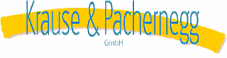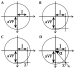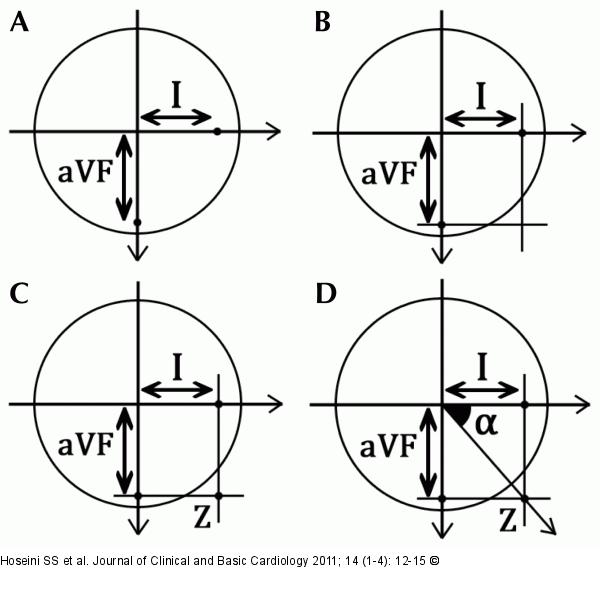Verlag für Medizin und Wirtschaft
Artikel   Bilder   Volltext

Mobile Version
A-  |   A  |   A+
Werbung

Hoseini SS, Moeeny A, Shoar S, Shoar N, Naderan M, Gharibzadeh SH, Dehpour AR
Designing Nomogram for Determining the Heart's QRS Axis
Journal of Clinical and Basic Cardiology 2011; 14 (1-4): 12-15

PDF    Summary    OverviewFigure/Graphic 1: QRS Complex
The drawing method for determination of the heart axis using the QRS complex. (a) Plot the net deflection of QRS complex (mean QRS) for both leads I and aVF along each lead; (b) Run perpendicular lines from each of the plotted points; (c) Z point represents the apex of the mean QRS axis; (d) Draw a vector between O point and Z point. The angle between lead I and OZ vector is the angle of the hearts electrical axis.Figure/Graphic 1: QRS ComplexThe drawing method for determination of the heart axis using the QRS complex. (a) Plot the net deflection of QRS complex (mean QRS) for both leads I and aVF along each lead; (b) Run perpendicular lines from each of the plotted points; (c) Z point represents the apex of the mean QRS axis; (d) Draw a vector between O point and Z point. The angle between lead I and OZ vector is the angle of the hearts electrical axis.

copyright © 2000–2023 Krause & Pachernegg GmbH | Sitemap | Datenschutz | Impressum